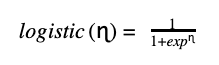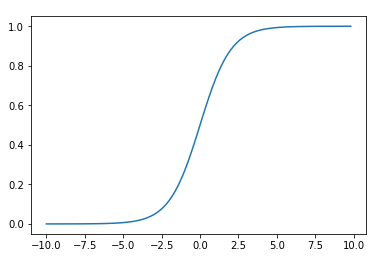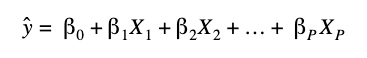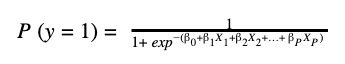# Logistic Regression

In machine learning, the Logistic Regression algorithm is used for classification problems. It provides an output that we can interpret as a probability that a new observation belongs to a certain class. Generally, logistic regression is used to classify binary classes but works on multiple and ordinal classes too.

Logistic regression estimates a continuous quantity which is the probability that an event occurs. This probability is compared  with a certain threshold that allow taking the decision about the classification of the new data.

If the threshold of the probability is equal to 0.5, we can classify the new data to each of the classes by comparing the probability value with that threshold.

Instead of fitting a straight line or hyperplane as in a linear regression model, the logistic regression model uses the logistic function to reflect the output of a linear equation between 0 and 1. With this function is possible to map real values of the predictions into probabilities. (Note: Logistic regression uses the sigmoid function for the two class logistic regression and the softmax function for the multiclass logistic regression).Logistic Regression Sigmoid FunctionThe logistic regression is a kind of reformulation of the linear regression for classification problems, as the linear regression is not good to separate classes. In a linear regression, we model the relationship between the features and the target variable with the following equation:In order to address classification problems, we convert this equation to obtain probabilities between 0 and 1. The right side of the above equation is wrapped into a logistic function, and therefore forces the output to be between 0 and 1.### Types of Logistic Regression

• Binary Logistic Regression: The target variable has only two possible outcomes.
• Multiple Logistic Regression: The target variable has three or more nominal categories.
• Ordinal Logistic Regression: The target variable has three or more ordinal categories such as assign movies score from 1 to 5.